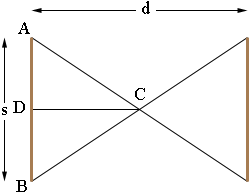SEARCH HOMEMath Central Quandaries & QueriesQuestion from mark, a parent: length of sides of an 18 ft .diameter bldg i need to find out if i should build an octagon or decagon to keep the sides of the bldg at 8ft or less. how do i figure the length of the sides of each?Hi Mark,

If you have a regular polygon with an even number of sides n then the opposite sides are parallel. In my diagram the distance between a pair of opposite sides is d units, you have d = 8 ft. I used s for the length of a side.Since the polygon is regular and the number of sides is n, the angle BCA has measure 360/n degrees. Thus the angle DCA has measure half of that which is 180/n degrees. Hence

tan(180/n) = |AD|/|DC| = (s/2)/(d/2) = s/d.

This gives the side length s as

s = d tan(180/n).

I hope this helps,
PennyMath Central is supported by the University of Regina and The Pacific Institute for the Mathematical Sciences.